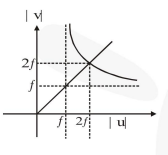# In an optics experiment,

Question:

In an optics experiment, with the position of the object fixed, a student varies the position of a convex lens and for each position, the screen is adjusted

to get a clear image of the object. A graph between the object distance $u$ and the image distance $v$, from the lens, is plotted using the same scale

for the two axes. A straight line passing through the origin and making an angle of $45^{\circ}$ with the $x$-axis meets the experimental curve at

$P$. The coordinates of $P$ will be :-

1. $(f, f)$

2. $(4 f, 4 f)$

3. $(2 f, 2 f)$

4. $\left(\frac{f}{2}, \frac{f}{2}\right)$

Correct Option: , 3

Solution: